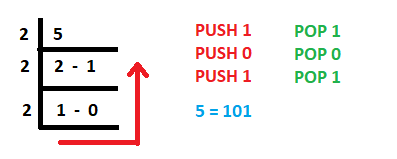# Java Program to Convert a Decimal Number to Binary Number using Stacks

Java is high level, compiled as well as interpreted programming language. Stack is an abstract data type used in most of the programming languages and can be implemented using arrays or linked list. Stack data structure follows the principle of LIFO (Last In First Out) . Stack allows push, pop, peek operations to be performed. The push operation is used to insert a new element into the stack. The pop operation is used to remove an element from the top of the stack. The peek operation is used to get the top element of the stack without removing it from the stack. A decimal number can be converted into binary number using the push and pop operation of the Stack. Now, Java provides inbuilt Stack class which can be used to suit our purpose.

Converting a decimal number to binary number using stacks:

1. Using predefined stack.
2. Using array as a stack.

Method 1: Using Predefined Stack

Approach:

• A predefined stack class is used to create a stack.
• The user is asked to enter a decimal number.
• Next, a while loop is executed where the mod result of the number by 2 is pushed into the stack and the number is divided by 2.
• This is repeated till the number is greater than 0.
• When the number is 0 the while loop is terminated and another while loop is starts to print the binary equivalent of the decimal number.
• The remainders stored in the stack are popped in LIFO which gives the desired binary representation.Code Implementation:

## Java

 `// Java Program to Convert a Decimal Number ` `// to Binary Number using Stacks`   `import` `java.util.*;` `public` `class` `DecimalToBinary` `{` `public` `static` `void` `main(String args[])` `{` `    ``// creating a stack ` `    ``Stack stack = ``new` `Stack();` `  `  `    ``System.out.println(``"Enter a decimal number: "``);` `    ``int` `num = ``23``;` `  `  `    ``while``(num>``0``)` `    ``{` `        ``int` `r = num%``2``;` `      `  `        ``// pushing the remainder inside the stack` `        ``stack.push(r);` `        ``num/=``2``;` `    ``}` `  `  `     ``System.out.print(``"Binary equivalent: "``);` `  `  `     ``while` `(!(stack.isEmpty() ))` `     ``{` `         ``// printing the resultant binary number stored in the ` `         ``// stack in LIFO manner` `         ``System.out.print(stack.pop());` `     ``}` `}` `}`

Output

```Enter a decimal number:
Binary equivalent: 10111```

The time complexity is O(log n), where n is the decimal number.

The auxiliary space is O(log n) as well.

Method 2: Using Array as a stack

Approach:

• The inbuilt stack class is not used rather an array is used and push and pop methods are defined to perform insertion and removal of the elements.
• The stack is implemented using the static array.
• The static variable count keeps track of the number of the elements inserted.
• The remaining procedure is exactly similar to the previous example.

## Java

 `// Java Program to Convert a Decimal Number` `// to Binary Number using Stacks`   `import` `java.util.*;` `public` `class` `DecimalToBinary {` `    ``static` `int` `arr[] = ``new` `int``[``1000``];`   `    ``// maintaining count variable as the top` `    ``// of the stack` `    ``static` `int` `count;`   `    ``// push at the count index and increment the count` `    ``public` `static` `void` `push(``int` `n) { arr[count++] = n; }`   `    ``// pop all the elements starting from count-1` `    ``// till 0` `    ``public` `static` `void` `pop()` `    ``{` `        ``for` `(``int` `i = count - ``1``; i >= ``0``; i--) {` `            ``System.out.print(arr[i]);` `        ``}` `    ``}`   `    ``public` `static` `void` `main(String args[])` `    ``{` `        ``System.out.println(``"Enter a decimal number: "``);` `        ``int` `num = ``46``;`   `        ``while` `(num > ``0``) {` `            ``int` `r = num % ``2``;` `            ``push(r);` `            ``num /= ``2``;` `        ``}`   `        ``System.out.print(``"Binary equivalent: "``);`   `        ``pop();` `    ``}` `}`

Output

```Enter a decimal number:
Binary equivalent: 101110```

Time complexity: O(logn) as here while loop runs logn times

Auxiliary space: O(1)

Whether you're preparing for your first job interview or aiming to upskill in this ever-evolving tech landscape, GeeksforGeeks Courses are your key to success. We provide top-quality content at affordable prices, all geared towards accelerating your growth in a time-bound manner. Join the millions we've already empowered, and we're here to do the same for you. Don't miss out - check it out now!

Previous
Next